# Introduction to Functional Data Analysis with R

by Joseph Rickert

Suppose you have data that looks something like this.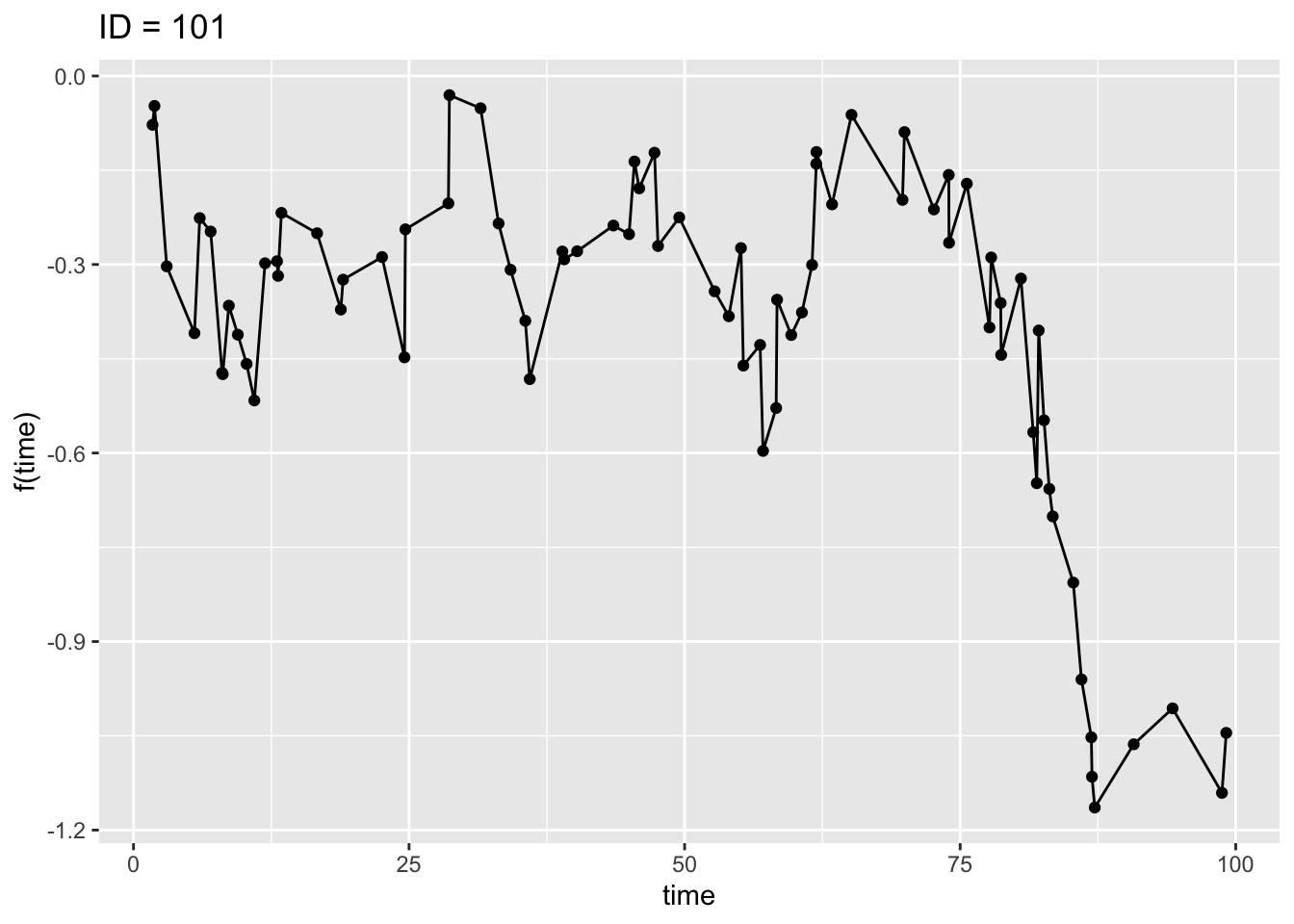This plot might depict 80 measurements for a participant in a clinical trial where each data point represents the change in the level of some protein level. Or it could represent any series of longitudinal data where the measurements are taken at irregular intervals. The curve looks like a time series with obvious correlations among the points, but there are not enough measurements to model the data with the usual time series methods. In a scenario like this, you might find Functional Data Analysis (FDA) to be a viable alternative to the usual multi-level, mixed model approach.

This post is meant to be a “gentle” introduction to doing FDA with R for someone who is totally new to the subject. I’ll show some “first steps” code, but most of the post will be about providing background and motivation for looking into FDA. I will also point out some of the available resources that a newcommer to FDA should find helpful.

FDA is a branch of statistics that deals with data that can be conceptualized as a function of an underlying, continuous variable. The data in FDA are smooth curves (or surfaces) in time or space. To fix a mental model of this idea, first consider an ordinary time series. For example, you might think of the daily closing prices of your favorite stock. The data that make up a time series are the individual points which are considered to be random draws from an underlying stochastic process.

Now, go up a level of abstraction, and consider a space where the whole time series, or rather an imaginary continuous curve that runs through all of your data points is the basic item of analysis. In this conceptual model, the curve comprises an infinite number of points, not just the few you observed. Moreover, unlike in basic time series analysis, the observed points do not need to be equally spaced, and the various curves that make up your data set do not need to be sampled at the same time points.

Mathematically, the curves are modeled as functions that live in an infinite dimensional vector space, what the mathematicians call a Hilbert Space. One way to think of this is that you are dealing with the ultimate large p small n problem. Each curve has infinitely many points, not just the 3 or 30 or 3,000 you happen to have.

The theory of Hilbert Spaces is part of the area of mathematical analysis called Functional Analysis, a subject usually introduced as part of a second or third course in mathematical analysis, or perhaps in a course on Quantum Mechanics.

It would be a heavy lift to expect someone new to Functional Data Analysis to start with the mathematics. Fortunately, this is not really necessary. The practical applications of FDA and the necessary supporting software have been sufficiently developed so that anyone familiar with the basics of ordinary vector spaces should have sufficient background to get started. The salient points to remember are:

• Hilbert space is an infinite dimensional linear vector space
• The vectors in Hilbert space are functions
• The inner product of two functions in the Hilbert space is defined as the integral of two functions, but it behaves very much like the familiar dot product.

Moreover, for the last twenty years or so mathematical statisticians have been writing R packages to put FDA within the reach of anyone with motivation and minimal R skills. The CRAN Task View on Functional Data Analysis categorizes and provides brief explanations for forty packages that collectively cover most of the established work on FDA. The following graph built with functions from the cranly package shows part of the network for two core FDA packages.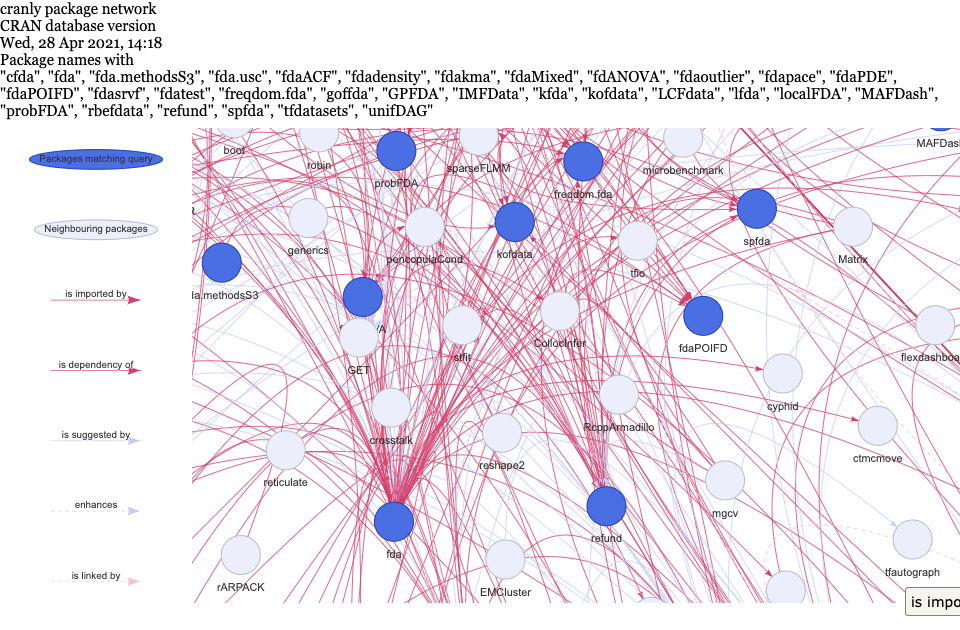The fda package emphasized in the network plot above is the logical place for an R user to begin investigating FDA. With thirty-two reverse depends, thirty-eight reverse imports and thirteen reverse suggest, fda is at the root of Functional Data Analysis software for R. Moreover, in a very real sense, it is at the root of modern FDA itself. fda was written to explicate the theory developed in the 2005 book by Ramsay and Silverman$$^{1}$$. Kokoszka and Reimnerr state that the first edition of this book published in 1997: “is largely credited with solidifying FDA as an official subbranch of statistics” (p xiv)$$^{2}$$. The refund package is used extensively throughout the book by Kokoszka and Reimnerr.

### First Steps

The synthetic data in the figure above were generated by a Wiener, Brownian Motion process, which for the purposes of this post, is just a convenient way to generate a variety of reasonable looking curves. We suppose that the data points shown represent noisy observations generated by a smooth curve f(t). We estimate this curve with the model: $$y_{i} = f(t_{i}) + \epsilon_{i}$$ where the $$\epsilon_{i}$$ are normally distributed with mean 0 and variance $$\sigma^{2}$$.

Notice that the measurement times are randomly selected within the 100 day window and not uniformly spaced.

set.seed(999)
n_obs <- 80
time_span <- 100
time <- sort(runif(n_obs,0,time_span))
Wiener <- cumsum(rnorm(n_obs)) / sqrt(n_obs)
y_obs <- Wiener + rnorm(n_obs,0,.05)

Remember that the task ahead is to represent the entire curve of infinitely many points and not just the handful of observed values. Here is where the linear algebra comes in. The curve is treated as a vector in an infinite dimensional vector space, and what we want is something that will serve as a basis for this curve projected down into the subspace where the measurements live. The standard way to do this for non-periodic data is to construct a B-spline basis. (B-splines or basis splines are splines designed to have properties that make them suitable for representing vectors.) The code that follows is mostly borrowed from Jiguo Cao’s Youtube Video Course$$^{3}$$ which I very highly recommend for anyone just starting with FDA. In his first five videos, Cao explains B-splines and the placement of knots in great detail and derives the formula used in the code to calculates the number of basis elements from the number of knots and the order of the splines.

Note that we are placing the knots at times equally spaced over the 100 day time span.

times_basis = seq(0,time_span,1)
knots    = c(seq(0,time_span,5)) #Location of knots
n_knots   = length(knots) #Number of knots
n_order   = 4 # order of basis functions: cubic bspline: order = 3 + 1
n_basis   = length(knots) + n_order - 2;
basis = create.bspline.basis(c(min(times_basis),max(times_basis)),n_basis,n_order,knots)
n_basis
##  23

and there are 23 basis vectors.

Next, we use the function eval.basis() to evaluate the basis functions at the times where our data curve was observed The matrix PHI contains the values of the 23 basis functions $$\phi_j(t)$$ evaluated at 80 points.

PHI = eval.basis(time, basis)
dim(PHI)
##  80 23

We plot the basis functions and locations of the knots.

matplot(time,PHI,type='l',lwd=1,lty=1, xlab='time',ylab='basis',cex.lab=1,cex.axis=1)
for (i in 1:n_knots)
{
abline(v=knots[i], lty=2, lwd=1)
}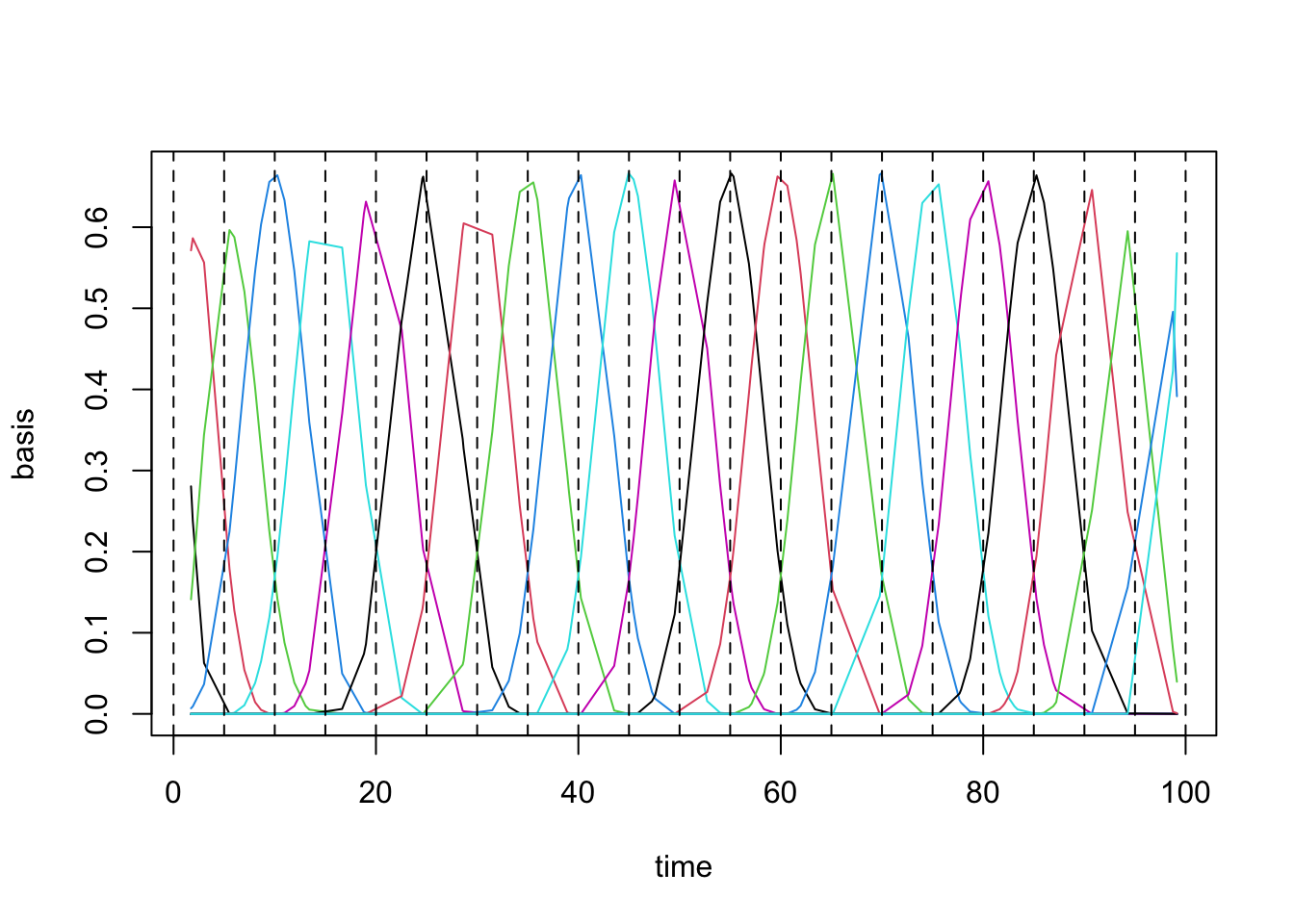The plot shows that for interior points, four basis functions contribute to computing the value of any point. The endpoints, however, are computed from a single basis function.

### Estimating the Basis Coefficients

As in ordinary regression, we express the function in terms of the coefficients $$c_j$$ and basis functions $$\phi_j$$ using the formula: $$f(t) = \sum c_j \phi_j(t)$$. Later we will see how to use built-in fda functions to estimate the coefficients, but now we follow Cao’s lead and calculate everything from first principles.

The following code uses matrix least squares equation $$\hat{c} = (\Phi^t\Phi)^{-1} \Phi^{t}y$$ to estimate the coefficients.

# Least squares estimate
# estimate basis coefficient
M = ginv(t(PHI) %*% PHI) %*% t(PHI)
c_hat = M %*% Wiener

We compute $$\hat{y}$$, the estimates of our observed values, and plot.

y_hat = PHI %*% c_hat
# Augment data frame for plotting
df <- df %>% mutate(y_hat = y_hat)
p2 <- df %>% ggplot() +
geom_line(aes(x = time, y = Wiener), col = "grey") +
geom_point(aes(x = time, y = y_obs)) +
geom_line(aes(x = time, y = y_hat), col = "red")
p2 + ggtitle("Original curve and least squares estimate") +
xlab("time") + ylab("f(time)")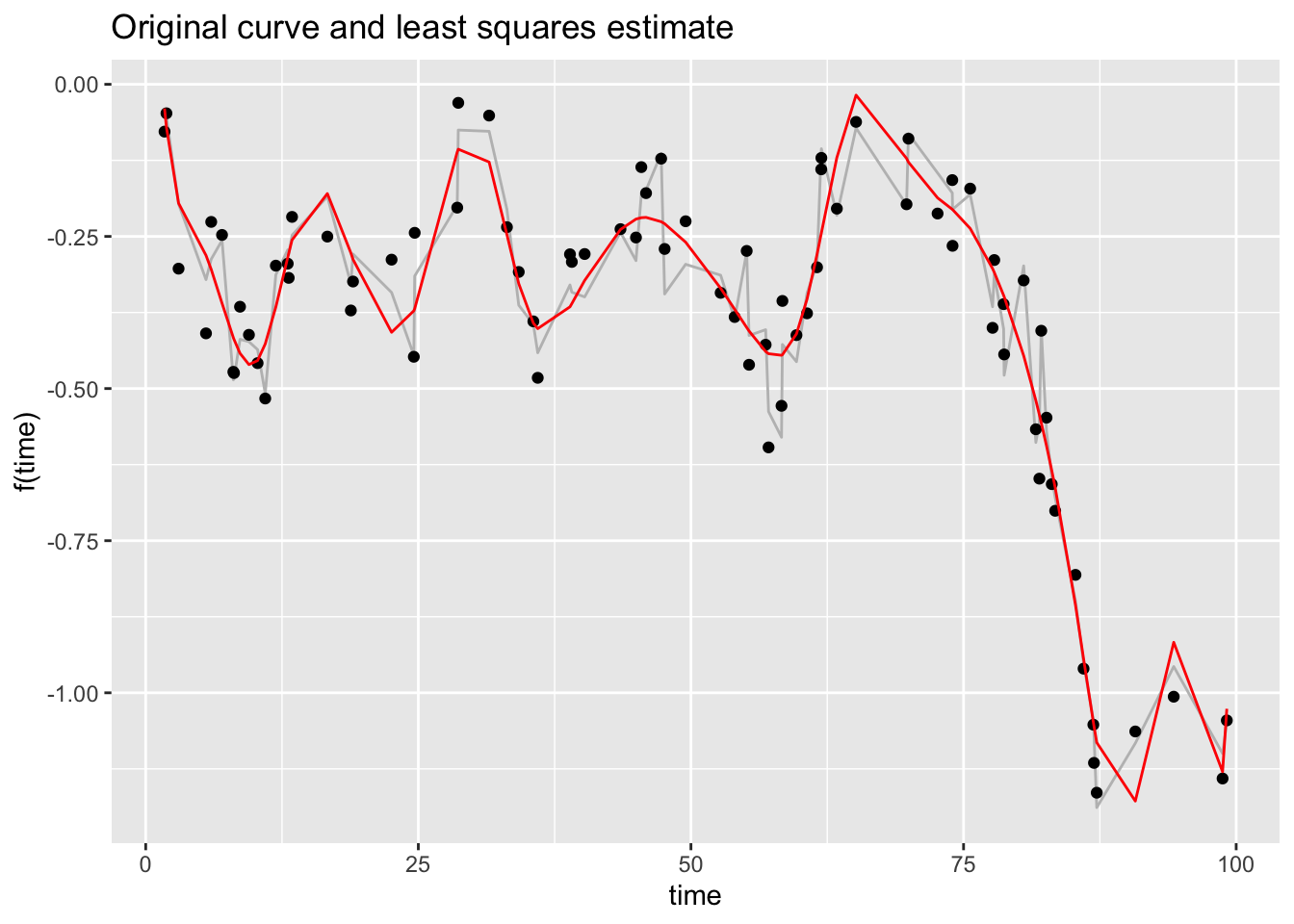The gray curve in the plot represents the underlying Brownian motion process, the dots are the observed values (the same as in the first plot), and the red curve represents the least squares “smoothed” estimates.

Now, we work through the matrix calculations to estimate the variance of the noise and the error bars for $$\hat{y}$$.

# estimate the variance of noise
## SSE = (Y - Xb)'(Y - Xb)
SSE = t(y_hat-y_obs)%*%(y_hat-y_obs)
sigma2 = SSE/(n_obs-n_basis)

# estimate the variance of the fitted curve
# H is the Hat matrix H
# H = X*inv(X'X)*X
H = PHI %*% M
varYhat = diag(H %*% H * matrix(sigma2,n_obs,n_obs))

# 95% confidence interval

y_hat025 = y_hat-1.96*sqrt(varYhat)
y_hat975 = y_hat+1.96*sqrt(varYhat)

And, we plot. We have a satisfying smoothed representation of our original curve that looks like it would be and adequate starting point for further study. Note that process of using regression to produce a curve from the basis functions is often referred to as “regression smoothing”

df <- mutate(df, y_hat025 = y_hat025,
y_hat975 = y_hat975)
#names(df) <- c("time","Wiener","y_hat", "y_hat025", "y_hat975")
p3 <- df %>% ggplot() +
geom_line(aes(x = time, y = Wiener), col = "grey") +
geom_point(aes(x = time, y = y_obs)) +
geom_line(aes(x = time, y = y_hat), col = "red") +
geom_line(aes(x = time, y_hat025), col = "green") +
geom_line(aes(x = time, y_hat975), col = "green")
p3 + ggtitle("Estimated curve with error bars") +
xlab("time") + ylab("f(time)")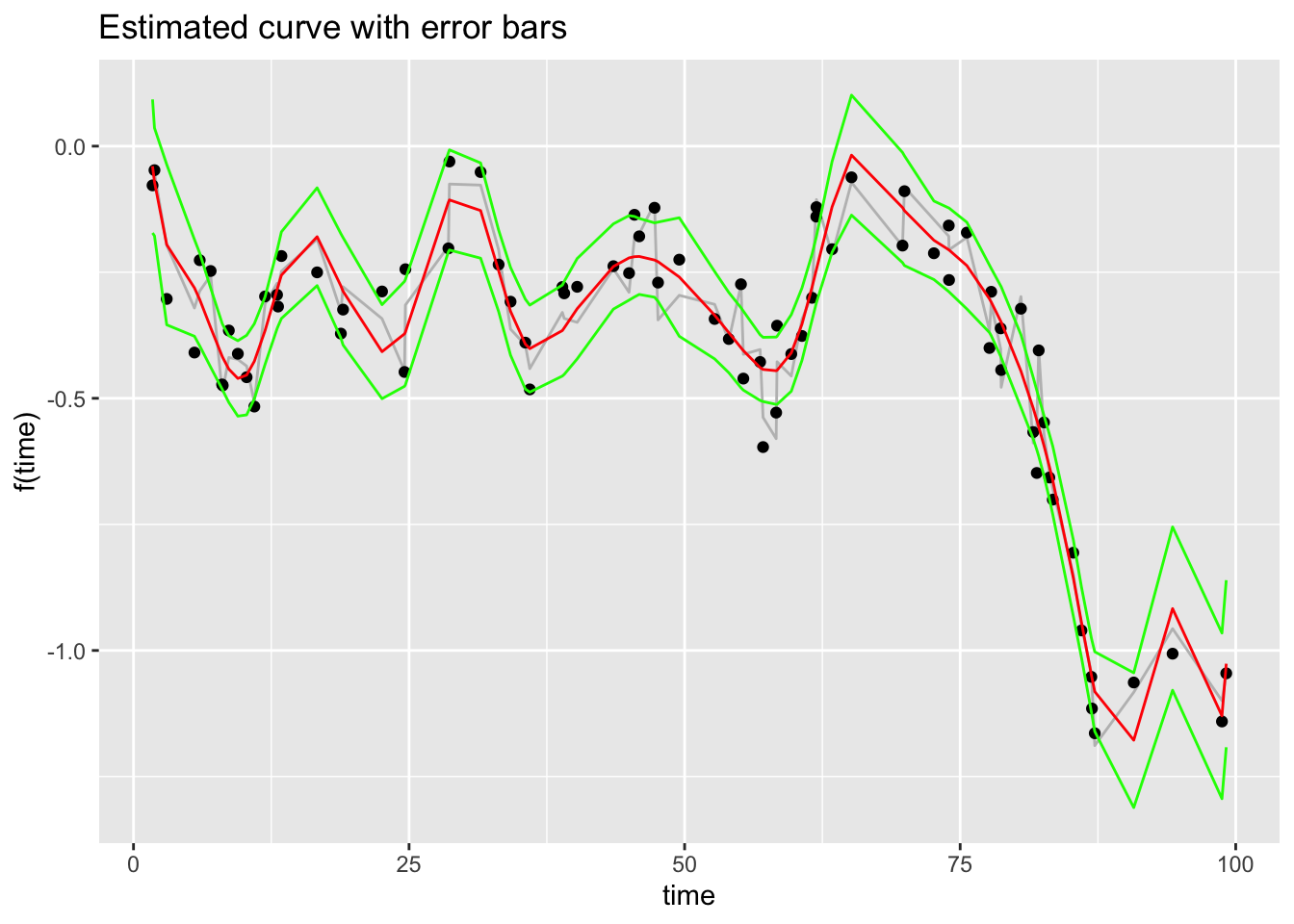We finish for today, by showing how to do the hard work of estimating coefficients and function values with a single line of code using the fda function smooth.basis(). The function takes the arguments argvals the times we want to use for evaluation as a vector (or matrix or array), y the observed values, and fdParobj, an fda object containing the basis elements.

Wiener_obj <- smooth.basis(argvals = time, y = y_obs, fdParobj = basis)

Here we plot our “hand calculated” curve in red and show the smooth.basis() curve in blue. They are reasonably close, except at the end points, where there is not much data to construct the basis.

plot(time, Wiener, type = "l", xlab = "time", ylab = "f(time)",
main = "Comparison of fda package and naive smoothing estimates", col = "grey")
lines(time,y_hat,type = "l",col="red")
lines(Wiener_obj, lwd = 1, col = "blue")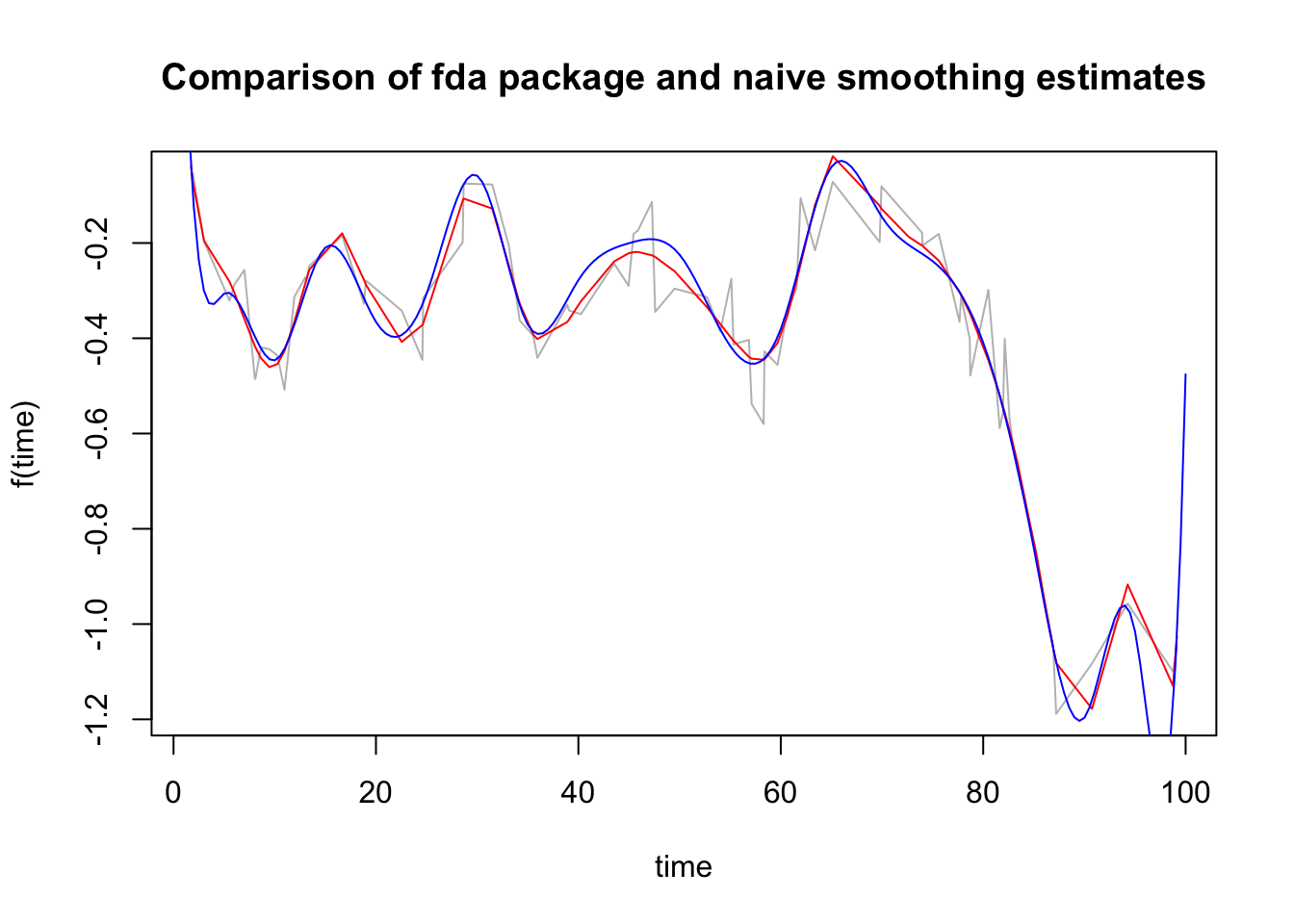Note that we have shown the simplest use of smooth.basis() which is capable of computing penalized regression estimates and more. The examples of using the smooth.basis() function in the fda pdf are extensive and worth multiple blog posts. In general, the pdf level documentation for fda is superb. However, the package lacks vignettes. For a price, the book Functional Data Analysis with R and Matlab$$^{4}$$ supplies the equivalent of several the missing vignettes.

### Next Steps

Once you have a basis representation, what’s next? You may be interested in the following:

• More exploratory work such as Functional Principal Components Analysis, the analog of principal components analysis.
• Clustering curves. See the funHDDC package.
• Setting up regression models where either the dependent variable, or some of the independent variables, or both are functional objects. See the refund package and the book by Kokoszka and Reimnerr$$^{2}$$
• Studying the shape of the curves themselves. For example, the shape of a protein concentration curve may convey some clinical meaning. FDA permits studying the velocity and acceleration of curves, offering the possibility of obtaining more information than the standard practice of looking at the area under the curves. You can explore this with the fda package (But be sure to check that the order of your basis functions is adequate to compute derivatives.). See the book by Ramsay, Hooker and Graves$$^{4}$$.
• Learning what to do when you have sparse data. See the paper by Yao et al. below$$^{5}$$ and look into the fdapace package.
• Working with two and three dimensional medical images$$^{6}$$.

I would like to make Functional Data Analysis a regular feature on R Views. If you are working with FDA and would like to post, please let me () know.